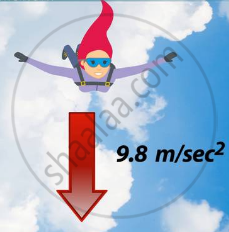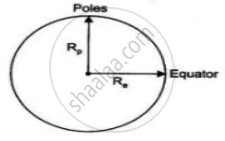Share

# Free Fall - Free Fall

#### notes

Free Fall:

Whenever objects fall towards the earth under this force alone, we say that the objects are in free fall. Is there any Whenever objects fall towards the earth under this force alone, we say that the objects are in free fall. Is there any But due to the earth’s attraction, there will be a change in the magnitude of the velocity. Any change in velocity involves acceleration. Whenever an object falls towards the earth, an acceleration is involved. This acceleration is due to the earth’s gravitational force. Therefore, this acceleration is called the acceleration due to the gravitational force of the earth (or acceleration due to gravity). It is denoted by g. The unit of g is the same as that of acceleration, that is, ms–2.As

F = ma             (becausea = g)  ...(1)

F = mg                                         ...(2)

and  "F" = "G"("Mm")/"d"^2    (therefore Universal law of gravitation)            ...(3)

From (ii) and (iii)

therefore  "mg" = "G"("Mm")/"d"^2

therefore  "g" = "GM"/"d"^2

M = Mass of the earth.

d = Distance between the object and the earth.

G = Gravitational constant

If the object is placed on the earth then d = R (radius of the earth)

therefore  "g" = "GM"/"R"^2

Earth is not a sphere it is flattened at poles.Hence R- Radius at pole and Re - Radius at equator   Re > Rp

"g" ∝ 1/"R"

therefore The value of 'g' is more at poles = (9.9 m/s2) and less at the equator = (9.8 m/s2)

### Shaalaa.com

Acceleration due to gravity [00:10:02]
S
series 1
0%

S